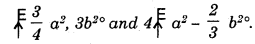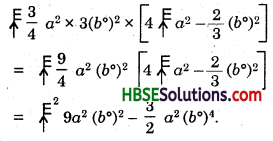# HBSE 8th Class Maths Solutions Chapter 9 Algebraic Expressions and Identities Ex 9.4

Haryana State Board HBSE 8th Class Maths Solutions Chapter 9 Algebraic Expressions and Identities Ex 9.4 Textbook Exercise Questions and Answers.

## Haryana Board 8th Class Maths Solutions Chapter 9 Algebraic Expressions and Identities Exercise 9.4

Question 1.
Multiply the binomials.
(i) (2x + 5) and (4x- 3)
(ii) (y – 8) and (3y – 4)
(iii) (2.5l – 0.5 m) and (2.5l + 0.5 m)
(iv) (a + 3b) and (x + 5)
(v) (2pq + 3q2) and 3(pq – 2q2)
(vi)Solution:
(i) (2x + 5) × (4x – 3)
= 8x2 – 6x + 20x – 15
= 8x2 + 14x – 15.

(ii) (y – 8) × (3y – 4) = 3y2 – 4y – 24y + 32
= 3y2 – 28y + 32.

(iii) (2.5l – 0.5m) × (2.5l + 0.5m)
= 6.25l2 + 1.25lm – 1.25lm + 0.25m2
= 6.25l2 + 0.25m2.

(iv) (a + 3b) × (x,+ 5)
= ax + 5a + 3bx + 15b
= ax + 3bx + 5a + 15b.(v) (2pq + 3q2) × (3pq – 2q2)
= 6p2q2 – 4pq3 + 9pq3 – 6q4
= 6p2a2 + 5pq3 – 6q4.

(vi)Question 2.
Find the product.
(i) (5 – 2x)(3 + x)
(ii) (x + 7y)(7x – y)
(iii) (a2 + b) (a + b2)
(iv) (p2 – q2) (2p + q).
Solution:
(i) (5 – 2x) × (3 + x)
= 15 + 5x – 6x – 2x2
= 15 – x – 2x2.

(ii) (x + 7y) × (7x -y)
= 7x2 – xy + 49xy – 7y2
= 7x2 – 7y2 + 48xy.(iii) (a2 + b) × (a + b2)
= a3 + a2b2 + ab + b3
= a3 + b3 + a2b2 + ab.

(iv) (p2 – q2) × (2p + q)
= 2p3 + p2q – 2pq2 – q3
= 2p3 – q3 + p2q – 2pq2.

Question 3.
Simplify :
(i) (x2 – 5) (x + 5) + 25
(ii) (a2 + 5) (b3 + 3) + 5
(iii) (t + s2) (t2 – s)
(iv) (a + b) (c – d) + (a – b) (c + d) + 2 (ac + bd)
(v) (x +y) (2x +y) + (x + 2y) (x – y)
(vi) (x + y) (x2 – xy + y2)
(vii) (1.5x – 4y) (1.5x + 4y + 3)- 4.5x + 12y
(viii) (a + b + c) (a + b – c).
Solution:
(i) (x2 – 5) × (x + 5) + 25
= x3 + 5x2 – 5x – 25 + 25
= x3 + 5x2 – 5x.(ii) (a2 + 5) × (b3 + 3) + 5
= a2b3 + 3a2 + 5b2 + 15 + 5
= a2b3 + 3a2 + 5b3 + 20.

(iii) (t + s2) (t2 – s)
= t3 – st + s2t2 – s3
= t3 – s3 + s2 + t2 – st.

(iv) (a + b) (c – d) + (a – b) (c + d) + 2 (ac +bd)
= ac – ad + bc – bd + ac + ad – bc – bd + 2ac + 2bd
= 4ac.

(v) (x + y) × (2x + y) + (x + 2y) x (x – y)
= 2x2 + xy + 2xy + y2 + x2 – xy + 2xy – 2y2
= 3x2 – y2 + 4xy.

(vi) (x + y) × (x2 – xy + y2)
= x3 – x2y + xy2 + x2y – xy2 + y3
= x3 + y3.(vii) (1.5x – 4y) × (1.5x + 4y + 3) – 4.5x + 12y
= 2.25x2 + 60xy + 4.5x – 60xy – 16y2 – 12y – 4.5x + 12y
= 2.25x2.

(viii) (a + b + c) × (a + b – c)
= a2 + ab – ac + ab + b2 – bc + ac + bc – c2
= a2 + b2 – c2 + 2ab.